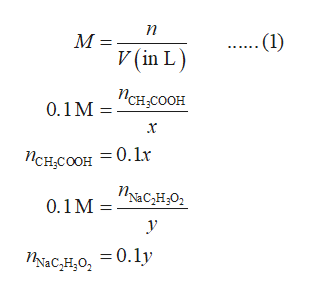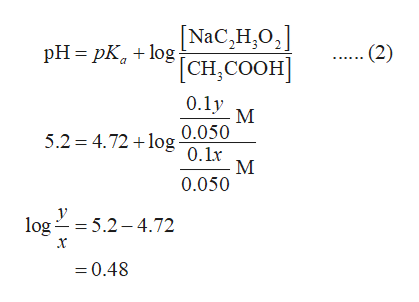# Given aqueous solutions of 0.1 M weak acetic acid (CH3COOH, pKa=4.72) and 0.1 M sodium acetate (NaC2H3O2), calculate the volumes of acid and acetate to be mixed, in order to make 50mL of a buffer solution with pH=5.2.

Question
75 views

Given aqueous solutions of 0.1 M weak acetic acid (CH3COOH, pKa=4.72) and 0.1 M sodium acetate (NaC2H3O2), calculate the volumes of acid and acetate to be mixed, in order to make 50mL of a buffer solution with pH=5.2.

check_circle

star
star
star
star
star
1 Rating
Step 1

Consider the volume of acetic acid in the final solution be x L and the volume of sodium acetate in the final solution be y L. The number of moles of both the species in their starting solutions can be calculated using equation (1),help_outlineImage Transcriptioncloseп - (1) M V (in L) Псн соон 0.1M = х = 0.1x Пснсоон Пасно 0.1M = Тласно, — 0.1у fullscreen
Step 2

The volume of the final buffer solution is 50 mL ( = 0.050 L).

The number of moles of acetic acid and sodium acetate in the final buffer solution can be calculated using equation (1),

Step 3

The relation between pH and pKa of a weak acid is given by the Hende...help_outlineImage Transcriptionclose|NaC,H,O, - (2) pH = pK, + log: [CH, СООН] 0.1y M 5.2 = 4.72 +log 0.050 0.1r 0.050 y log = 5.2 – 4.72 х = 0.48 fullscreen

### Want to see the full answer?

See Solution

#### Want to see this answer and more?

Solutions are written by subject experts who are available 24/7. Questions are typically answered within 1 hour.*

See Solution
*Response times may vary by subject and question.
Tagged in

### Chemistry